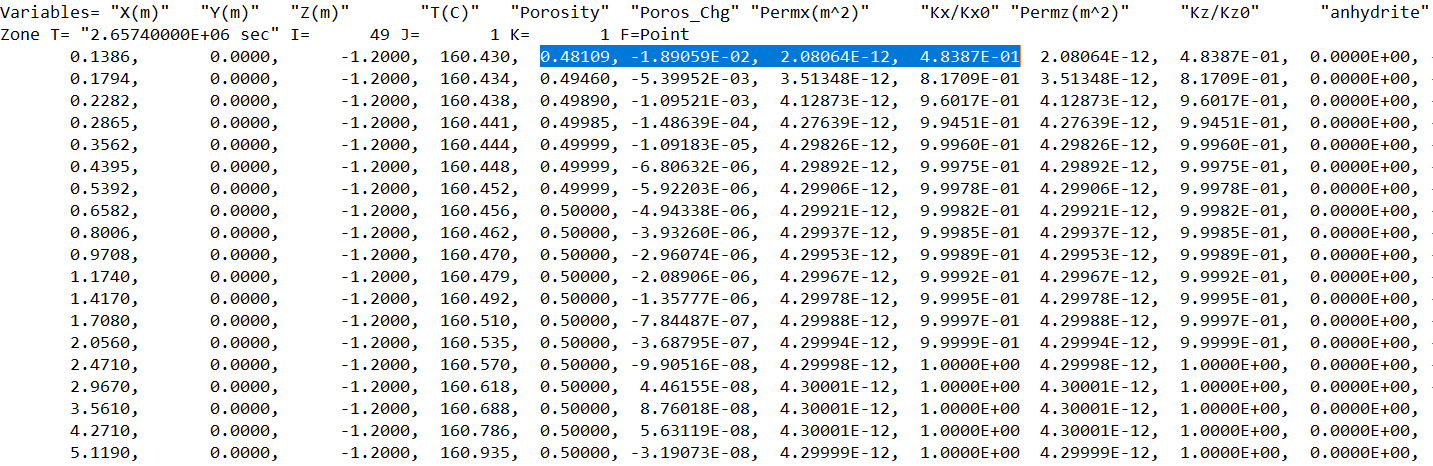0# Permeability-Porosity relationI use the TOUGHREACT EOS1 to model chemical reactions in porous media. In the Permeability-Porosity relation, I choose the Verma-Pruess correlation. The equation is k/ki=(phi-phi_c/phi_i-phi_c)^n.

In the model, I choose the phi_c as 0.46 and the n as 10. However, the simulation result doesn't match the correlation and the chosen parameters.

The initial porosity is 0.5, the critical porosity is 0.46 and the exponent is 10. The expected k/ki is 0.00166, however, the simulation result is 0.48387. I wonder if I miss another keyword causing this issue.

3replies Oldest first
• Oldest first